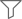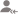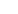trueAll

Lessons

DiscussionLesson Posted on 20/08/2018 SymmetryRaj Kumar

I am Six Sigma Black belt trained from American Society of Quality I am 2011 pass out in B.tech from...

Symmetry is nothing but Quality of being made of the precisely similar parts Nature loves symmetry Now we will check the symmetry 1) if the curve is symmetrical about the x-axis a)Y will be replaced by (-y), and curve remains the same for example, y2=x represents the parabola which is symmetrical... read more

Symmetry is nothing but Quality of being made of the precisely similar parts

Nature loves symmetry

Now we will check the symmetry

1)

if the curve is symmetrical about the x-axis

a)Y will be replaced by (-y), and curve remains the same

for example, y2=x represents the parabola which is symmetrical about the x-axisproof: replace y with -y in the above equation

(-y)2=x⇒y2=x so the curve remains same

2)

if the curve is symmetrical about the y-axis

a) f(-x)=f(x) means curve should be even function

for example, x2=y represents the parabola which is symmetrical about the y-axis

proof: replace x with -x in the above equation

(-x)2=y⇒x2=y so the curve remains same

(-x)2=y⇒x2=y so the curve remains same3)

if the curve is symmetrical about the line y=x

on interchanging of x and y Curve remains the same

For example, Cubic function y=x3 is symmetrical about the line y=x

let us interchange the x and y

⇒x=y3

Solve for y=x1/3 below given graph are two graphs which are symmetrical about the line y=xDislike Bookmark ShareSujoy D.

Tutor

NOSum of all the angles of a triangle is 180.As 70+115 is exceeding 180 degrees, it is not possible to construct a triangle.
Dislike Bookmark ShareSujoy D.

Tutor

Draw a straight 6 cm line. Then take compass and by taking 6cm in compass draw an arc from one of the line which you have drawn and without disturbing the compass from the other end of the line cut the arc which is drawn before. There you will get a point and now from that point join the ends of the... read more

Draw a straight 6 cm line. Then take compass and by taking 6cm in compass draw an arc from one of the line which you have drawn and without disturbing the compass from the other end of the line cut the arc which is drawn before. There you will get a point and now from that point join the ends of the line which is of 6 cm. Now equilateral triangle is done.

Dislike Bookmark Share

Overview

Questions 16

Total Shares33 FollowersSujoy D.

Tutor

English alphabets having symmetry are: A, B, C, D, E, K, M, T, U, V, W, Y have one line of symmetry. H, I, X have two lines of symmetry. And F, G, J, L, N, P, Q, R, S, Z have neither horizontal nor vertical lines of symmetry.
Dislike Bookmark ShareSujoy D.

Tutor

Cone.
Dislike Bookmark ShareSujoy D.

Tutor

An edge
Dislike Bookmark ShareSujoy D.

Tutor

2 edges.
Dislike Bookmark ShareVikas Verma

Tutor

Vertices.
Dislike Bookmark ShareTapas Bhattacharya

Tutor

Dislike Bookmark ShareAnkit

JEE/Board Maths Tutor

First draw a base line of 7 cm. Then take a measurement in compass of 4 cm using scale. Draw an Arc from one of the end point of the base line. Repeat the same procedure for 5 cm from another end point of base line. Draw two lines to both ends from the mid point of the Arc.
Dislike Bookmark Share

UrbanPro.com helps you to connect with the best in India. Post Your Requirement today and get connected.

x

X

### Find Tutors, Trainers & Institutes near you

Post requirement and connect with the tutors in your locality

• Post a learning requirement
• Get customized responses
• Compare and select the best### Want to learn something New?

Find best tutors, trainers & institutes near you on UrbanProUrbanPro.com is India's largest network of most trusted tutors and institutes. Over 25 lakh students rely on UrbanPro.com, to fulfill their learning requirements across 1,000+ categories. Using UrbanPro.com, parents, and students can compare multiple Tutors and Institutes and choose the one that best suits their requirements. More than 6.5 lakh verified Tutors and Institutes are helping millions of students every day and growing their tutoring business on UrbanPro.com. Whether you are looking for a tutor to learn mathematics, a German language trainer to brush up your German language skills or an institute to upgrade your IT skills, we have got the best selection of Tutors and Training Institutes for you. Read more